Home > A2C > Chapter 7 > Lesson 7.1.2 > Problem7-28

7-28.
1. Given f(x) = −2x2 − 4 and g(x) = 5x + 3, calculate: Homework Help ✎

1. g(−2)

2. f (−7)

3. f (g(−2))

4. f (g(1))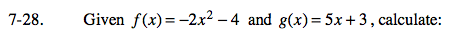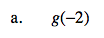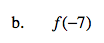Substitute the given value for x into the indicated equation.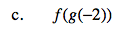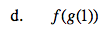Substitute the entire g(−2) into the equation f(x) for x and evaluate. Follow the same guidelines for part (d).